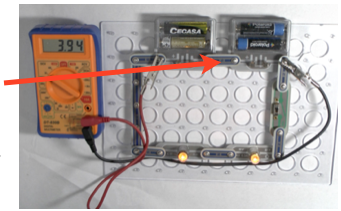Potential Difference and energy notes and video lesson

The potential difference (PD)  is measuring the level of energy delivered to or delivered by the current. The potential difference (PD)  measured in volts across the power supply measures the energy in joules given to each coulomb.If the PD is one volt then the cell provides each coulomb with one joule of energy so:

Energy = charge x PD

and since charge = current x time

then

energy = current x time x PD

in symbols

E = ItV

Where E is in joules, I in amps, t in seconds and V in volts

Here the potential difference is measuring the power provided by the current to the lamp. If the PD is one volt then  each coulomb provides the lamp with one joule of energy so:

The equations are exactly the same, again:

Energy = charge x PD

and since charge = current x time

then  energy = current x time x PD

in symbols  E = ItV

In this case the two values of PD are the same since the only component in the circuit apart from the cell is the lamp. Almost all the energy leaving the cell is transferred to the lamp since only a tiny amount of energy is used to drive the current through the thick copper wires.

Some energy will however be changed to heat inside the cell- see internal resistance and EMF.

Measuring the potential difference across a lamp

Electrical energy and power

Since power = energy transferred/time

Then electrical power = ItV/t

so Power = IV